# GRE Subject Test: Math : Eigenspace Classifications

## Example Questions

### Example Question #1 : Eigenvalues

Find the eigenvalues of the following matrix, if possible.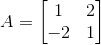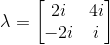The eigenvalues do not exist.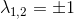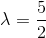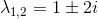Explanation:

In order to find the eigenvalues of a matrix, apply the following formula: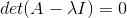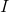is the identity matrix.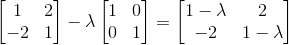Compute the determinant and set it equal to zero.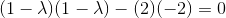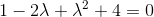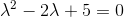Solve for lambda by using the quadratic formula.### Example Question #2 : Eigenvalues

Find the eigenvalues of the following matrix, if possible.The eigenvalues do not exist.Explanation:

In order to find the eigenvalues of a matrix, apply the following formula:is the identity matrix.Compute the determinant and set it equal to zero.Solve for lambda by using the quadratic formula.### All GRE Subject Test: Math Resources